# Colleges with the lowest SAT scores in Minnesota

Top 10 colleges in Minnesota with the lowest SAT scores
Looking for the colleges with the lowest SAT scores in Minnesota? Well you're in luck! We've compiled a national college database and have created a list of the top 10 universities with the lowest SAT scores in Minnesota below. If you are not a good test taker or worried about your test scores, this list is for you. These are the schools whose applicants had the lowest average SAT scores in Minnesota, which means that you can get into these colleges with a lower SAT score. We also include each college's ACT scores and acceptance rate so that you can see where you would have the easiest time getting in. Read on to find out more.

## Southwest Minnesota State University SAT scores

The average SAT score for Southwest Minnesota State University is 990.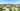The average SAT score of 990 breaks down into:

• SAT math: 490

The average ACT score for Southwest Minnesota State University is 21 and their acceptance rate is 80%.

## Bethel University SAT scores

The average SAT score for Bethel University is 1058.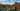The average SAT score of 1058 breaks down into:

• SAT math: 520

The average ACT score for Bethel University is 22 and their acceptance rate is 89.8%.

## Minnesota State University Moorhead SAT scores

The average SAT score for Minnesota State University Moorhead is 1060.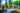The average SAT score of 1060 breaks down into:

• SAT math: 540

The average ACT score for Minnesota State University Moorhead is 23 and their acceptance rate is 60.3%.

## Concordia University Saint Paul SAT scores

The average SAT score for Concordia University Saint Paul is 1080.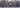The average SAT score of 1080 breaks down into:

• SAT math: 540

The average ACT score for Concordia University Saint Paul is 21 and their acceptance rate is 54.9%.

## Saint Cloud State University SAT scores

The average SAT score for Saint Cloud State University is 1080.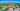The average SAT score of 1080 breaks down into:

• SAT math: 540

The average ACT score for Saint Cloud State University is 21 and their acceptance rate is 85.1%.

## Bemidji State University SAT scores

The average SAT score for Bemidji State University is 1110.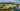The average SAT score of 1110 breaks down into:

• SAT math: 555

The average ACT score for Bemidji State University is 22 and their acceptance rate is 66.2%.

## Minnesota State University Mankato SAT scores

The average SAT score for Minnesota State University Mankato is 1110.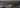The average SAT score of 1110 breaks down into:

• SAT math: 555

The average ACT score for Minnesota State University Mankato is 22 and their acceptance rate is 61%.

## Augsburg College SAT scores

The average SAT score for Augsburg College is 1130.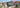The average SAT score of 1130 breaks down into:

• SAT math: 540

The average ACT score for Augsburg College is 22 and their acceptance rate is 45%.

## Crown College SAT scores

The average SAT score for Crown College is 1140.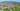The average SAT score of 1140 breaks down into:

• SAT math: 560

The average ACT score for Crown College is 22 and their acceptance rate is 56.2%.

## Saint Mary's University of Minnesota SAT scores

The average SAT score for Saint Mary's University of Minnesota is 1140.The average SAT score of 1140 breaks down into:

• SAT math: 570